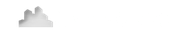### Modeling Detailed Expense Stops or Base Years

Sometimes, an Expense or Base Year Stop is specific to certain expenses.

LeaseMatrix does allow for itemized Expense Stops. Below is one such example.

In this instance, there is \$2.00 Expense Stop on Electricity, with the Landlord passing thru any overages. Here is what we input:

Step 3: Expense AssumptionsRather than using the Expense Stop input, we'll need to detail out this expense line item for our tenant. In doing so we enter:

#1. Electricity Pass Thrus. This amount is assumed to be equal to the Expense Stop in this instance at \$2.00 per RSF

#2. The Pass Thru Inflation Rate. We assume the cost of Electricity will grow at an Inflation rate of 3.00% per year. This inflation rate represents the overage of the Electricity expense which will ultimately be passed thru to the Tenant.

#3. Electricity Base Year. This -\$2.00 per RSF amount could represent the estimated Base Year or a specified Expense Stop. Note this input number is negative. This is because the Landlord is the one paying for this amount, so it is deducted from what the Tenant's actual Electricity usage is.

#4. The Base Year Inflation Rate. Input the rate of 0.00% here, because this line item is the Base Year input, so, by definition, this number does not increase.

Once these inputs are complete, we can click Save & Preview. Here is the result:Notice how, in Year 1, the Electricity Pass Thru amount of \$22,400 is offset 100% by the Electricity Base Year of -\$22,400. Then in Year 2, \$672 is Passed Thru to the Tenant. (eg. \$22,400 * 3.0% inflation = \$672.00).

This level of detail is a great way to use LeaseMatrix's detailed reports to help your tenant understand exactly what an Expense Stop or Base Year Stop is.

(If they need further explaination, this article may help.)

## Alter the timing of when a Base Year begins.

It is possible to alter when the Base Year Stop begins.

For instance, if the lease starts in May 2014 and the Base Year is established using 2014's final expenses, beginning in 2015, you can trigger the start date of this Expense Stop and Pass Thru combination by specifying that the each line item begins in month 9 (i.e. Month 9 = January of 2015).

If you need to delay the timing of the inflation rate of an expense, you may do so using this calculator.